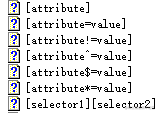# JQuery 获取元素和选择器用法以及属性的介绍

## 选择器用法

*$("*")所有元素 #id$("#lastname")id="lastname" 的元素
.class$(".intro")所有 class="intro" 的元素 element$("p")所有 <p> 元素
.class.class$(".intro.demo")所有 class="intro" 且 class="demo" 的元素 :first$("p:first")第一个 <p> 元素
:last$("p:last")最后一个 <p> 元素 :even$("tr:even")所有偶数 <tr> 元素
:odd$("tr:odd")所有奇数 <tr> 元素 :eq(index)$("ul li:eq(3)")列表中的第四个元素（index 从 0 开始）
:gt(no)$("ul li:gt(3)")列出 index 大于 3 的元素 :lt(no)$("ul li:lt(3)")列出 index 小于 3 的元素
:not(selector)$("input:not(:empty)")所有不为空的 input 元素 :header$(":header")所有标题元素 <h1> - <h6>
:animated所有动画元素

:contains(text)$(":contains('W3School')")包含指定字符串的所有元素 :empty$(":empty")无子（元素）节点的所有元素
:hidden$("p:hidden")所有隐藏的 <p> 元素 :visible$("table:visible")所有可见的表格

s1,s2,s3$("th,td,.intro")所有带有匹配选择的元素 [attribute]$("[href]")所有带有 href 属性的元素
[attribute=value]$("[href='#']")所有 href 属性的值等于 "#" 的元素 [attribute!=value]$("[href!='#']")所有 href 属性的值不等于 "#" 的元素
[attribute$=value]$("[href$='.jpg']")所有 href 属性的值包含以 ".jpg" 结尾的元素 :input$(":input")所有 <input> 元素
:text$(":text")所有 type="text" 的 <input> 元素 :password$(":password")所有 type="password" 的 <input> 元素
:radio$(":radio")所有 type="radio" 的 <input> 元素 :checkbox$(":checkbox")所有 type="checkbox" 的 <input> 元素
:submit$(":submit")所有 type="submit" 的 <input> 元素 :reset$(":reset")所有 type="reset" 的 <input> 元素
:button$(":button")所有 type="button" 的 <input> 元素 :image$(":image")所有 type="image" 的 <input> 元素
:file$(":file")所有 type="file" 的 <input> 元素 :enabled$(":enabled")所有激活的 input 元素
:disabled$(":disabled")所有禁用的 input 元素 :selected$(":selected")所有被选取的 input 元素
:checked$(":checked")所有被选中的 input 元素 ###$("#id属性") id选择器

$(function () {$("#btn").click(function () {
});
});

$(".cls").css("border", "1px solid red"); //设置某个元素的css样式属性值 .css("属性","值") 复制代码 ###$("标签名字") 标签选择器

//页面加载的事件
$(function () { //根据id选择器获取按钮,添加点击事件$("#btn").click(function () {
//根据标签选择器获取p标签
$("p").text("我们都是p标签"); }); }); .text()方法相当于DOM中的.innerText属性 对象.text() -> 获取该元素的文本内容 对象.text("值") -> 设置该元素的文本内容 复制代码 ⚠️注意: 本身代码没有循环操作，jQuery中内部帮助我们循环操作的 -> 隐式迭代 ###$(标签名.类样式的名字) 交集选择器（标签+类选择器）

$(function () {$("#btn").click(function () {
$("p.cls").css("backgroundColor", "green"); }); }); 复制代码 ###$(选择器,选择器..) 并集选择器（多条件选择器）

$(function () {$("#btn").click(function () {
$("#dv,p,.cls").css("backgroundColor", "orange"); }); }); 复制代码 ### 层次选择器（后代选择器） $("A B") 后代选择器        //选择A元素内部的所有B元素
$("A > B") 子选择器 //选择A元素内部的所有B子元素$("#A~B") 后面的全部元素   //获取的是A这个父级元素后面的所有的兄弟元素（B）
$("#A+B") 下一个元素 //获取的是div后面直接的兄弟元素$("#btn").click(function () {
// 获取的是div这个父级元素中所有span标签(直接的子元素,孙子元素)
$("#div span").css("backgroundColor","red"); // 获取的是div这个父级元素中直接的子元素$("#div>span").css("backgroundColor","red");

// 获取的是div这个父级元素后面的所有的兄弟元素---span
$("#div~span").css("backgroundColor","red"); // 获取的是div后面直接的兄弟元素$("#div+span").css("backgroundColor","red");
});

### 属性选择器

$("A[属性名]") 属性名称选择器 //包含指定属性的选择器$("A[属性名='值']") 属性值选择器   //包含指定属性等于指定值的选择器
$("A[属性名='值'][]...") 复合属性选择器 //包含多个属性条件的选择器 复制代码### 过滤选择器（筛选器） $("选择器:first")          首元素选择器    //多元素集合的第一个元素
$("选择器:last") 尾元素选择器 //多元素集合的最后一个元素$("选择器:odd")            奇数选择器
$("选择器:even") 偶数选择器$("选择器:lt(索引)")        小于索引选择器  //获取小于这个索引的元素
$("选择器:gt(索引)") 大于索引选择器 //获取大于这个索引的元素$("选择器:header")         标题选择器      //获得标题（h1~h6）元素，固定写法
$("选择器:eq(index)") 等于索引选择器 //$('div').eq(5);   //选择第6个div元素
$("选择器:not(selector)") 非元素选择器 //$('div').not('.myClass'); //选择不等于myClass的div元素

表单过滤选择器
:enabled   获得可用元素
:disabled  获得不可用元素
:checked   获得单选/复选框选中的元素
:selected  获得下拉框选中的元素

.index()           索引选择器      //元素的索引

// 操作当前元素集，删除不匹配的元素，得到一个新的集合
.filter()
$('li').filter('.a').css('background-color', 'red') // 检索该条件在每个元素的后代中是否存在，将符合条件的的元素构成新的结果集 .has()$('li').has('span').css('background-color', 'red')

// 当前选中元素的上下文中找到符合条件的后代，返回的是子元素
.find()
$("ul>li:odd").css("backgroundColor", "yellow"); //0dd -> 奇数的 </script> 复制代码 ### 链式编程 <script src="https://libs.baidu.com/jquery/2.1.1/jquery.min.js"></script> <ul> <li>1</li> <li>2</li> <li>3</li> <li>4</li> </ul> <script>$(function () {
$("ul>li").mouseenter(function () {$(this).css("backgroundColor", "red")
}).mouseleave(function () {
$(this).css("backgroundColor", "") }).click(function () {$(this).css("color", "green").css("fontSize", "30px").css("fontFamily", "全新硬笔行书简")
})
})
</script>

### 验证用户输入

<script src="https://libs.baidu.com/jquery/2.1.1/jquery.min.js"></script>

<script>
$(function () {$("input[name=username]").blur(function () {
var pattern = /^[\u4e00-\u9fa5]{4,8}$/; if (pattern.test($(this).val())) {
$(this).css("background", "green") } else {$(this).css("background", "red") }
});
})
</script>